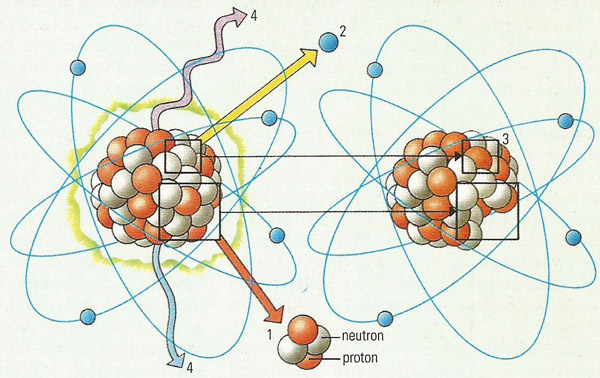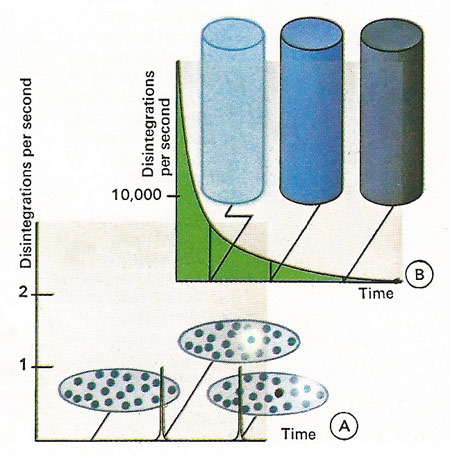A# DarlingMany elements are naturally radioactive, so there is always background radiation even when there is no man-made or accidentally generated radioactivity in the vicinity. Alpha radiation occurs when an unstable atomic nucleus emits an alpha (1) particle, a helium nucleus comprising of two protons and two neutrons. Alpha radiation (α) is stopped by flesh. Beta radiation (β) occurs when an electrically neutral neutron emits a negative electron (which is the beta (2) particle) and becomes in the process a positive proton (3). Beta particles can be stopped by a thin sheet of aluminum. X-rays and gamma rays (γ) are both electromagnetic radiation (types of light), created when a nucleus loses energy without undergoing structural change (4). X-rays can be stopped by a sheet of lead, while high-energy gamma rays will penetrate thin sheets of lead, but may be stopped by a layer of concrete.Radioactive atoms have a certain chance of decaying which is not affected by the presence or absence of other atoms. The rate of disintegration of a radioactive material is proportional to the amount of radioactive substance present multiplied by the chance that an atom will decay. Whilst we cannot say when a single radioactive atom will decay [A], if ever, we can forecast the average behavior of a number of radioactive atoms [B]. Electrons, gamma rays or helium nuclei can be emitted.

Radioactivity is the spontaneous breaking apart, or decay, of unstable atomic nuclei. The three types of radioactive emission are alpha particles (nuclei of helium, which corresponds to a decrease in mass of the nucleus by four units), beta particles (fast-moving electrons, which corresponds to an increase in atomic number of the nucleus by one unit), and gamma rays (high-energy photons, which corresponds to a loss in energy by the nucleus). Radioactive nuclei are formed in supernova explosions. Radioactivity is measured in units of becquerels or disintegrations per second. See also activity and ionizing radiation.

There are less common types of radioactive decay. Positron emission results in a decrease by one unit in the atomic number; K capture involves the incorporation of one of the extranuclear electrons into the nucleus, the atomic number is again decreased by one unit.

Radioactivity was discovered for uranium by Henri Becquerel in 1896. If the substances, or to be more precise, the radionuclides occur in nature, one refers to natural radioactivity; if they are a product of nuclear conversions in nuclear reactors or accelerators, one refers to artificial radioactivity. More than 2,750 radionuclides are known today. Each radionuclide is characterized by its half-life, the time during which half the atomic nuclei convert in a given quantity. Half-lives of several billion years (uranium-238; even still longer-lived is tellurium-128 with a half-life of 7.2 × 1024 years) to the millionth of a second (Po-212) are known. The radiation emitted during decay and its energy is also characteristic. For example, radium-226 decays emitting alpha radiation, while iodine-131 emits beta rays.

## Decay constant

The decay constant (λ) of radioactive decay is equal to the reciprocal value of the average lifetime (τ). Between the decay constant, the average lifetime, and the half-life (T½) the following relations exist:

λ = τ -1 = T½-1 · ln 2